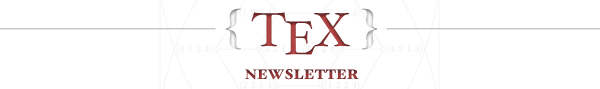## Top new questions this week:

### Plot Dini's surface

According to Wikipedia, the Dini's surface is described by the following parametric equations: x = a \cos u \sin v y = a \sin u \sin v z = a (\cos v + \ln\tan v/2) + bu So, I'd like to plot the ...

pgfplots pstricks plot tikz-3dplotasked by Sigur 20 votesanswered by Schrödinger's cat 19 votes

### Why does the hyperref documentation suggest using gather instead of equation?

On p39 of the hyperref documentation, there is a rather cryptic suggestion to use gather instead of equation. "the situation for equation is unclear, because nobody is interested in ...

hyperrefasked by Howard 14 votesanswered by David Carlisle 20 votes

### A sad duck with TikZ (or TikZducks?)

I am working on my homework, which includes a little website. I like the tikzducks package very much, so I decided to add some illustrations there using the svg files generated from \duck command. ...

tikz-pgf tikzducks tikzlingsasked by Someone 8 votesanswered by Ulrike Fischer 15 votes

### Is there a way to draw a simple circle or square in plain TeX/LaTeX, without relying on graphics packages?

I need to draw some bullets for a custom list, but what I have now is pgf code. I figured, why load entire pgf package to draw a mere circle (or square) when there must be (don't tell me otherwise, ...

circles shapes bulletsasked by bp2017 8 votesanswered by David Carlisle 12 votes

### How come the catcode of ' is 12 while ' is let to be a macro?

' is defined in latex.ltx as follows \def\active@math@prime{^\bgroup\prim@s} {\catcode`\'=\active \global\let'\active@math@prime} \def\prim@s{% \prime\futurelet\@let@token\pr@m@s} \def\pr@m@s{% ...

math-mode macros tex-coreasked by Symbol 1 7 votesanswered by Steven B. Segletes 10 votes

### ConTeXt: specify a path to font in \definefontfamily

I'm very new to ConTeXt, so please excuse the newbie question. I'm trying to load a font from a file. The .otf files are stored in a directory named fonts/ which is in the directory of the .tex file ...

fonts contextasked by Kresimir 6 votesanswered by Kresimir 2 votes

### Custom hatched pattern imitating hand drawing

Following this great question, I have been drawing a picture that simulates hand drawing. I want to fill some solids with a simple hatch pattern, however I don't know how to apply the path decorator ...

tikz-pgf patternasked by jsb 6 votesanswered by Schrödinger's cat 10 votes

## Greatest hits from previous weeks:

### Column and row padding in tables

How do you guys go about controlling column padding in tables? Update To clarify, I'm looking for a way to control both vertical and horizontal padding. So, if I had a simple table like in the ...

tables spacing columnsasked by JustDanyul 310 votesanswered by Werner 272 votes

### What is the difference between \dfrac and \frac?

The title says it all, both \dfrac{a}{b} and \frac{a}{b} make fractions, so what is the difference between the two?

math-mode amsmathasked by YYC 109 votes

### Using \footnote in a figure's \caption

Maybe this is an easy one, but I struggled with this now too long :) I want to have a footnote in a caption of a figure, see the example. \begin{figure}[!ht] \caption{a figure ...

floats footnotes captionsasked by Matten 228 votesanswered by Dee 163 votes

### Change line spacing inside the document

This is my code \documentclass[12pt,a4paper]{book} \renewcommand{\baselinestretch}{1.5} \begin{document} \include{Chapter1} \end{document} I have please a question: I would change the line ...

line-spacingasked by researcher 100 votes

### Scale image to page width?

I use \inclugraphics[scale=TRYING TO GUESS THE NUMBER FOR PAGE WIDTH]{...} but I find this errorsome, is there some ready flag to set the image to page width? I usually use PNG and JPG photos.

graphics scalingasked by hhh 198 votesanswered by Werner 200 votes

### How can I get bold math symbols?

To make Latin-letter variables bold I can use e.g. \mathbf{a}, but while putting Greek letters or symbols such as \nabla inside \mathbf doesn't cause any errors or warnings, it also doesn't do ...

fonts math-mode symbols boldasked by Michael Underwood 422 votesanswered by Mark Meckes 372 votes

### Align equation left

Here is my split equation: \documentclass{article} \usepackage{amsmath} \begin{document} \begin{equation} \begin{split} a_{ijk} =& \frac {Pr(M_{I} =2 \& M_J=1 \& M_K =1 | I=i , J=j , ...

equations alignasked by user35571 55 votesanswered by Ludovic C. 59 votes

## Can you answer these questions?

### How to debug token positions in petri nets in TikZ?

Following an example given in How to make this TikZ picture more idiomatic? (environment diagram) I tried to use the pre-defined "pic" to place more "function definition icons" on the canvas using ...

tikz-pgf tikz-picasked by Vladimir Nikishkin 1 voteanswered by Schrödinger's cat 0 votes

### Beamer class identifies table as figure when using subcaption

The Tex Version in my office was recently updated and I am having trouble getting everything back in order. Specifically, I want to use the subcaption package, which seems to mark tables as figures. ...

beamer cleverefasked by dumbledore 1 vote

### Can't get a line on a graph from a .csv file

I am trying to generate a plot from a .csv file. My .csv file contains the following data and it is named HeightsAndWeights.csv: To generate the plot I use the following code: ...

tikz-pgf axisasked by Chris Kirov 1 vote
You're receiving this message because you subscribed to the TeX - LaTeX community digest.
Unsubscribe from this community digest       Edit email settings       Leave feedback       PrivacyStack Overflow, 110 William Street, 28th floor, New York, NY 10038

 <3